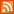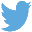Bucaro TecHelpHTTPS Encryption not required because no account numbers orpersonal information is ever requested or accepted by this siteCustom Search# Rounding a Number with JavaScript

The result of a JavaScript mathematical operation can be a number with up to 16 digits. To make this result more human readable you might want to round it to 3 or 4 digits. To accomplish this you could use the JavaScript Math object's .round method, as shown below.

```Math.round(317.47);
```

The .round method follows the convention of rounding numbers with the decimal .5 and higher to the next higher integer, and numbers with decimals lower than .5 to the next lower integer. So the example shown above would return 317.

In some cases you may need to round the result to a certain number of didts to the right of the decimal point. You can acomplish this with the function shown below.

```function roundDecimal(number, digits)
{
// shift the decimal point to the right
var a = number * Math.pow(10,digits);

// round the shifted number
var b = Math.round(a);

// shift the decimal point back
var c = b / Math.pow(10,digits);

return c;
}
```

Calling the above function as shown below returns 317.473.

```roundDecimal(317.47285, 3);
```

If your mathematical operation is being performed on currency, the easiest way to format the result is to use the JavaScript .toFixed method, which rounds a number to the specified number of digits to the right of the decimal point, and converts it to a string. You can acomplish this with the function shown below.

```function toCurrency(amount)
{
var dols = "\$ " + amount.toFixed(2);
return dols;
}
```

Calling the above function as shown below returns \$ 987.40.

```toCurrency(987.4);
```

The .round method follows the convention of rounding numbers with the decimal .5 and higher to the next higher integer, and numbers with decimals lower than .5 to the next lower integer. If you want to always round up, regardless of the value of the numbers to the right of the decimal point, use the object's .ceil method, as shown below.

```Math.ceil(317.47);
```

The example shown above would return 318.

If you want to always round down, regardless of the value of the numbers to the right of the decimal point, use the object's .floor method, as shown below.

```Math.floor(317.67);
```

The example shown above would return 317.

 .menubtn { margin-top:10px; margin-left:50px; width:220px; opacity:1; border-style:solid; border-color:#009000; background-color:#66ff66; transition-property: opacity; transition-duration: 1s; transition-timing-function: ease-out; } .menubtn:hover { opacity:1; background-color:#e5ff23; } Menu - More Java Script Quick ReferenceRSS FeedFollow @Stephen Bucaro[Site User Agreement] [Privacy Policy] [Site map] [Search This Site] [Contact Form]
Copyright©2001-2023 Bucaro TecHelp 13771 N Fountain Hills Blvd Suite 114-248 Fountain Hills, AZ 85268